# AP Statistics : How to find descriptive data from a z-score

## Example Questions

### Example Question #1 : How To Find Descriptive Data From A Z Score

There are four suspects in a police line-up, and one of them committed a robbery. The suspect is described as "abnormally tall". In this case, "abnormally" refers to a height at least two standard deviations away from the average height. Their heights are converted into the following z-scores:

Suspect 1: 2.3

Suspect 2: 1.2

Suspect 3: 0.2

Suspect 4: -1.2.

Which of the following suspects committed the crime?

Suspect 4

Suspect 1

Suspect 3

Suspect 2

Suspect 1

Explanation:

Z-scores describe how many standard deviations a given observation is from the mean observation. Suspect 1's z-score is greater than two, which means that his height is at least two standard deviations greater than the average height and thus, based on the description, Suspect 1 is the culprit.

### Example Question #1 : Graphing Data

A value has a-score of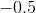.  The value is . . .

below the population mean

two standard deviations from the population mean

the same as the population mean

one standard deviation from the population mean

above the population mean

below the population mean

Explanation:

The-score indicates how close a particular value is to the population mean and whether the value is above or below the mean.  A positive-score is always above the mean and a negative-score is always below it.  Here, we know the value is below the mean because we have a negative-score.

### Example Question #2 : How To Find Descriptive Data From A Z Score

All of the students at a high school are given an entrance exam at the beginning of 9th grade. The scores on the exam have a mean ofand a standard deviation of. Sally's z-score is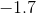. What is her score on the test?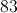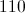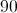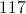Explanation:

The z-score equation is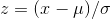.

To solve forwe have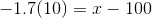.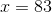### Example Question #3 : How To Find Descriptive Data From A Z Score

Your professor gave back the mean and standard deviation of your class's scores on the last exam.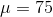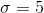Your friend says the z-score of her exam is.

What did she score on her exam?Explanation:

The z-score is the number of standard deviations above the mean.

We can use the following equation and solve for x.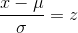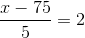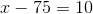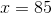Two standard deviations above 75 is 85.### Example Question #4 : How To Find Descriptive Data From A Z Score

Your boss gave back the mean and standard deviation of your team's sales over the last month.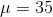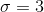Your friend says the z-score of her number of sales is.

How many sales did she make?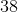Explanation:

The z-score is the number of standard deviations above or below the mean.

We can use the known information with the following formula to solve for x.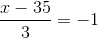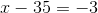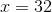### Example Question #5 : How To Find Descriptive Data From A Z Score

The following data set represents Mr. Marigold's students' scores on the final. The standard deviation for this data set is 8.41. If you scored 0.91 standard deviations worse than the mean, what was your score?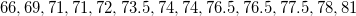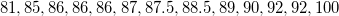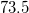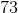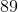Explanation:

To work with a z-score, first we need to find the mean of the data set. By adding together and dividing by 26, we get 81.15.

We know that your score is 0.91 standard deviations WORSE than the mean, which means that your z-score is -0.91. We can use the following formula for the z-score: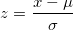where z is the z-score, x is your data point,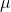is the mean, 81.15, and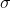is the standard deviation, which we are told is 8.41.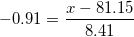multiply both sides by 8.41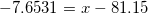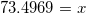we can reasonably round this to 73.5, which is an actual score in the data set. That must be your grade.

### Example Question #6 : How To Find Descriptive Data From A Z Score

Your teacher gives you the z-score of your recent test, and says that the mean score was a 60, with a standard deviation of 6. Your z-score was a -2.5. What did you score on the test?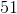Explanation:

To find out your scoreon the test, we enter the given information into the z-score formula and solve for.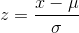whereis the z-score,is the mean, andis the standard deviation.

As such,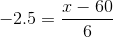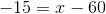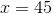So you scored aon the test.